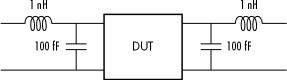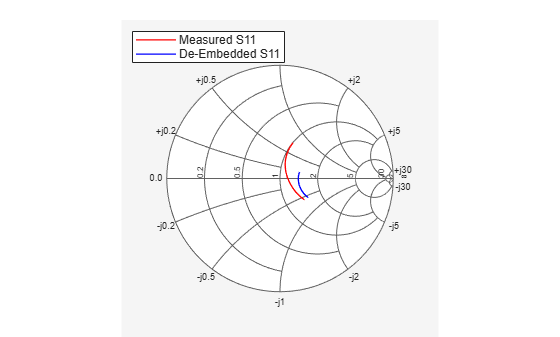# De-Embedding S-Parameters

This example shows you how to extract the S-parameters of a Device Under Test (DUT). First, read a Touchstone® file into a `sparameters` object, calculate the S-parameters for the left and right pads, de-embed the S-parameters using the `deembedsparams` function and then display the results.

This example will use the S-parameter data in the file `samplebjt2.s2p` that was collected from a bipolar transistor in a fixture with a bond wire (series inductance 1 nH) connected to a bond pad (shunt capacitance 100 fF) on the input, and a bond pad (shunt capacitance 100 fF) connected to a bond wire (series inductance 1 nH) on the output, see Figure 1.Figure 1: Device under test (DUT) and the test fixture.

This example will show how to remove the effects of the fixture in order to extract the S-parameters of the DUT.

Create an `sparameters` object for the measured S-parameters, by reading the Touchstone® data file `samplebjt2.s2p`.

```S_measuredBJT = sparameters('samplebjt2.s2p'); freq = S_measuredBJT.Frequencies;```

### Calculate S-Parameters for the Left Pad

Create a two port `circuit` object representing the left pad, containing a series `inductor` and shunt `capacitor`. Then calculate the S-parameters using the frequencies from `samplebjt2.s2p`.

```leftpad = circuit('left'); add(leftpad,[1 2],inductor(1e-9)) add(leftpad,[2 3],capacitor(100e-15)) setports(leftpad,[1 3],[2 3]) S_leftpad = sparameters(leftpad,freq);```

### Calculate S-Parameters for the Right Pad

Create a two port `circuit` object representing the right pad, containing a series `inductor` and shunt `capacitor`. Then calculate the S-parameters using the frequencies from `samplebjt2.s2p`.

```rightpad = circuit('right'); add(rightpad,[1 3],capacitor(100e-15)) add(rightpad,[1 2],inductor(1e-9)) setports(rightpad,[1 3],[2 3]) S_rightpad = sparameters(rightpad,freq);```

### De-Embed the S-Parameters

De-embed the S-parameters of the DUT from the measured S-parameters by removing the effects of input and output pads (`deembedsparams`).

`S_DUT = deembedsparams(S_measuredBJT,S_leftpad,S_rightpad);`

### Plot the Measured and De-Embedded S11 Parameters on a Z Smith® Chart

Use `smithplot` to plot the measured and de-embedded S11 parameters.

```figure; hs = smithplot(S_measuredBJT,1,1); hold on smithplot(S_DUT,1,1); hs.ColorOrder = [1 0 0; 0 0 1]; hs.LegendLabels = {'Measured S11','De-Embedded S11'};```### Plot the Measured and De-Embedded S22 Parameters on a Z Smith Chart

Use `smithplot` to plor the measured and de-embedded S22 parameters.

```hold off smithplot(S_measuredBJT,2,2); hold on smithplot(S_DUT,2,2); hs = smithplot('gco'); hs.ColorOrder = [1 0 0; 0 0 1]; hs.LegendLabels = {'Measured S22','De-Embedded S22'};```

### Plot the Measured and De-Embedded S21 Parameters in Decibels

Use `rfplot` to plot the measured and de-embedded S21 parameters.

```hold off h1 = rfplot(S_measuredBJT,2,1,'-r'); hold on h2 = rfplot(S_DUT,2,1); legend([h1,h2],{'Measured S_{21}','De-Embedded S_{21}'})```